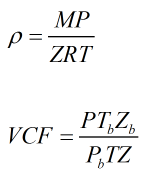# C256 – Gas Density Computation PTZ

## Description

This calculates either the line or standard density depending on the option selected.

Kelton calculation reference C256

FLOCALC calculation reference F065

KIMS calculation reference K205

## Options

### Solve for

• Line density
• Standard density

Select option based on which density you wish to calculate.

### Calculate

• Relative density

Allows user to calculate the output density as a relative density.

### Calculate from

• Standard density
• Relative density
• Molecular weight
• Line density

Select option based on the data you wish to enter.

## Constants

Molar gas constant – R = 8.314621 Jmol-1K-1

## Calculation

The calculation uses the following pressure, temperature and compressibility relationships to calculate line or standard density.Where M = Molelcular weight P = Pressure Pb = Pressure at base conditions T = Temperature Tb = Temperature at base conditions Z = Compressibility factor Zb = Compressibility factor at base conditions

Back